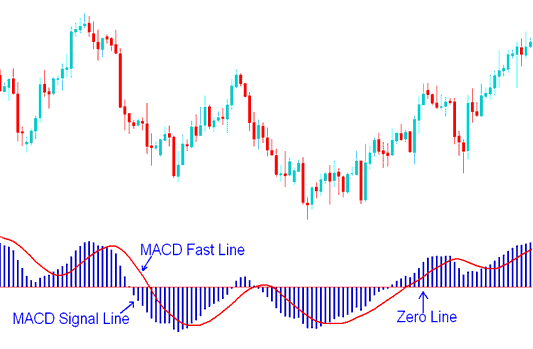# MACD Indicator Oscillator Forex Technical Analysis Fast Line and Signal Line

MACD Forex indicator is used in various ways to give technical analysis information.

1. MACD center line crosses indicate bullish or bearish markets; below zero is bearish, above zero is bullish.
3. Oscillations can be used to indicate oversold and overbought regions
4. Used to look for divergence between price and indicator.

## Construction of MACD Forex Indicator

The MACD forex indicator is constructed using two exponential moving averages and this forex technical indicator plots two lines. The two default exponential moving averages used are 12 and 26. Then a smoothing factor of 9 is also applied when drawing the MACD forex indicator.

Summary of how MACD forex indicator is drawn

MACD uses 2 EMAs + a smoothing factor (12, 26 Exponential Moving Averages and 9 smoothing periods)

MACD forex technical indicator only plots two lines - the MACD fast line and the MACD signal lineMACD Lines - MACD Fast Line and MACD Signal Lines Forex Trading Signals

• The Fast Line is the difference between the 26 EMA and 12 EMA
• The Signal line is the 9 period moving average of the MACD fast line.

## Implementation of MACD Forex Indicator

MACD forex indicator implements the MACD line as a continuous line while the signal line is implemented as a histogram. These two MACD LINES are then used to generate forex trading signals using the crossover trading strategy method.

There is also the MACD center-line which is also known as the zero mark and it is a neutral point between buyers and sellers trading the forex market.

Values above the center-mark are considered bullish forex signals while those below are bearish forex signals.

The MACD forex indicator being an oscillator indicator, oscillates above and below this center line.

Forex Contest Limited Time